# 5 Concepts You Should Know About Gradient Descent and Cost Function

Why is Gradient Descent so important in Machine Learning? Learn more about this iterative optimization algorithm and how it is used to minimize a loss function.This picture was taken at the top of Brick Hill (Nam Long Shan, Hong Kong).

Gradient descent is an iterative optimization algorithm used in machine learning to minimize a loss function. The loss function describes how well the model will perform given the current set of parameters (weights and biases), and gradient descent is used to find the best set of parameters. We use gradient descent to update the parameters of our model. For example, parameters refer to coefficients in Linear Regression and weights in neural networks.

In this article, I’ll explain 5 major concepts of gradient descent and cost function, including:

• Reason for minimising the Cost Function
• The calculation method of Gradient Descent
• The function of the learning rate
• Batch Gradient Descent (BGD)
• Stochastic gradient descent (SGD)

# What is the Cost Function?

The primary set-up for learning neural networks is to define a cost function (also known as a loss function) that measures how well the network predicts outputs on the test set. The goal is to then find a set of weights and biases that minimizes the cost. One common function that is often used is the mean squared error, which measures the difference between the actual value of y and the estimated value of y (the prediction). The equation of the below regression line is hθ(x) = θ + θ1x, which has only two parameters: weight (θ1)and bias (θ0).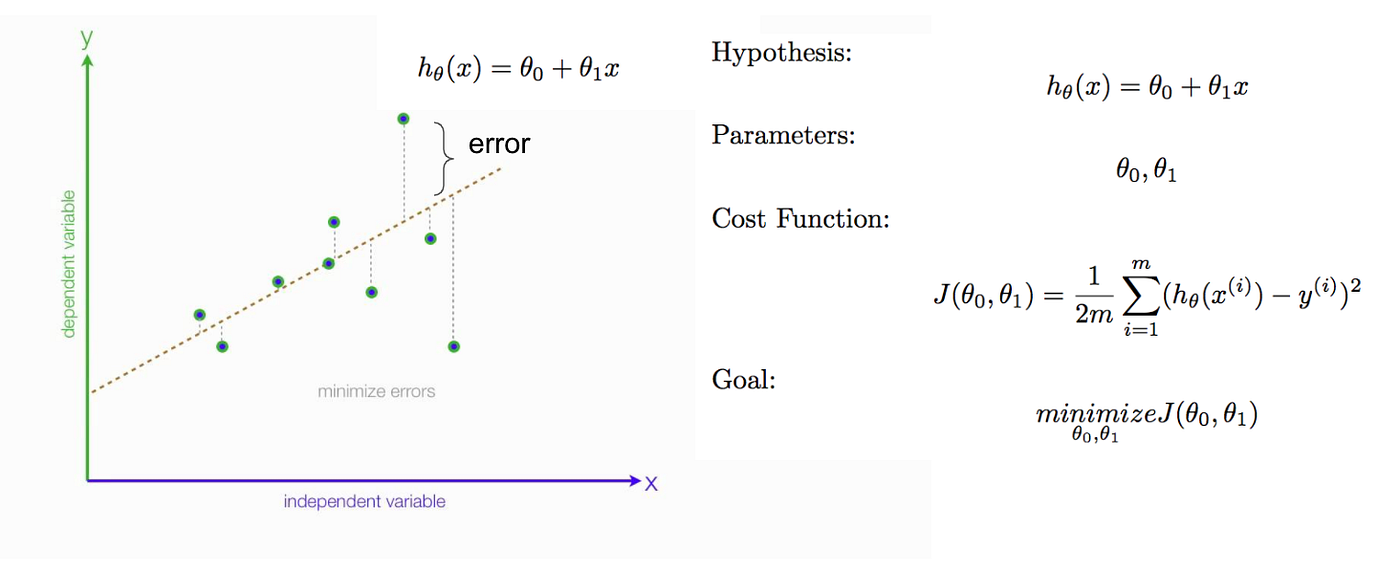## Minimising Cost function

The goal of any Machine Learning model is to minimize the Cost Function.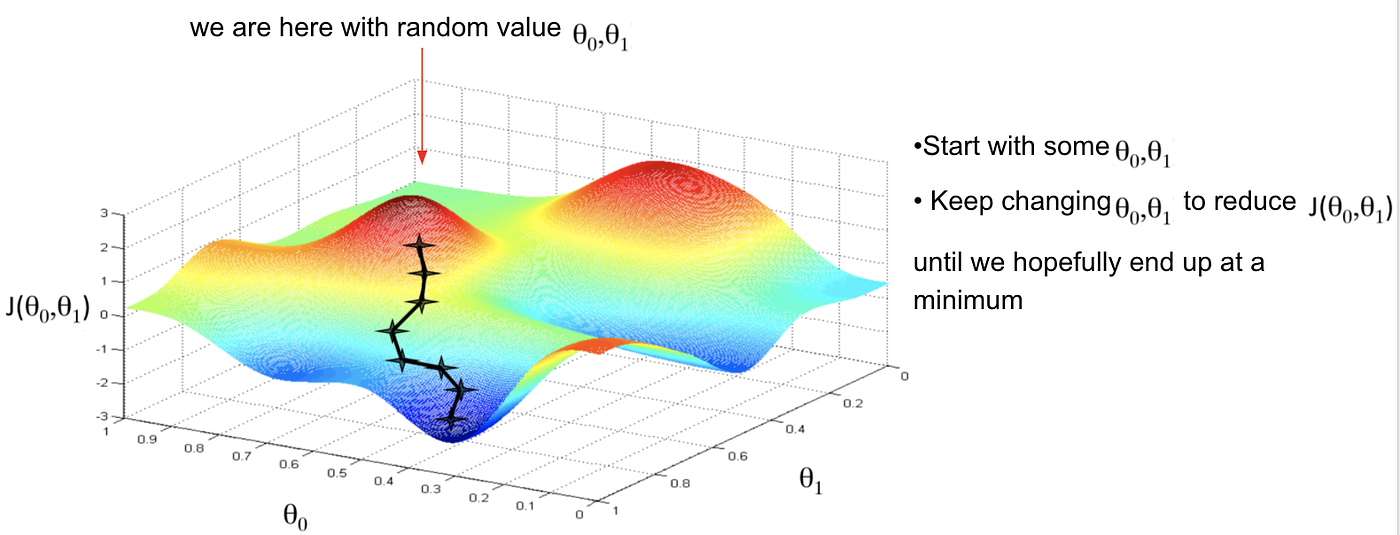# How to Minimise the Cost Function

Our goal is to move from the mountain in the top right corner (high cost) to the dark blue sea in the bottom left (low cost). In order to get the lowest error value, we need to adjust the weights ‘θ0 and ‘θ1’ to reach the smallest possible error. This is because the result of a lower error between the actual and the predicted values means the algorithm has done a good job in learning. Gradient descent is an efficient optimization algorithm that attempts to find a local or global minimum of a function.

## Calculating gradient descent

Gradient Descent runs iteratively to find the optimal values of the parameters corresponding to the minimum value of the given cost function, using calculus. Mathematically, the technique of the ‘derivative’ is extremely important to minimise the cost function because it helps get the minimum point. The derivative is a concept from calculus and refers to the slope of the function at a given point. We need to know the slope so that we know the direction (sign) to move the coefficient values in order to get a lower cost on the next iteration.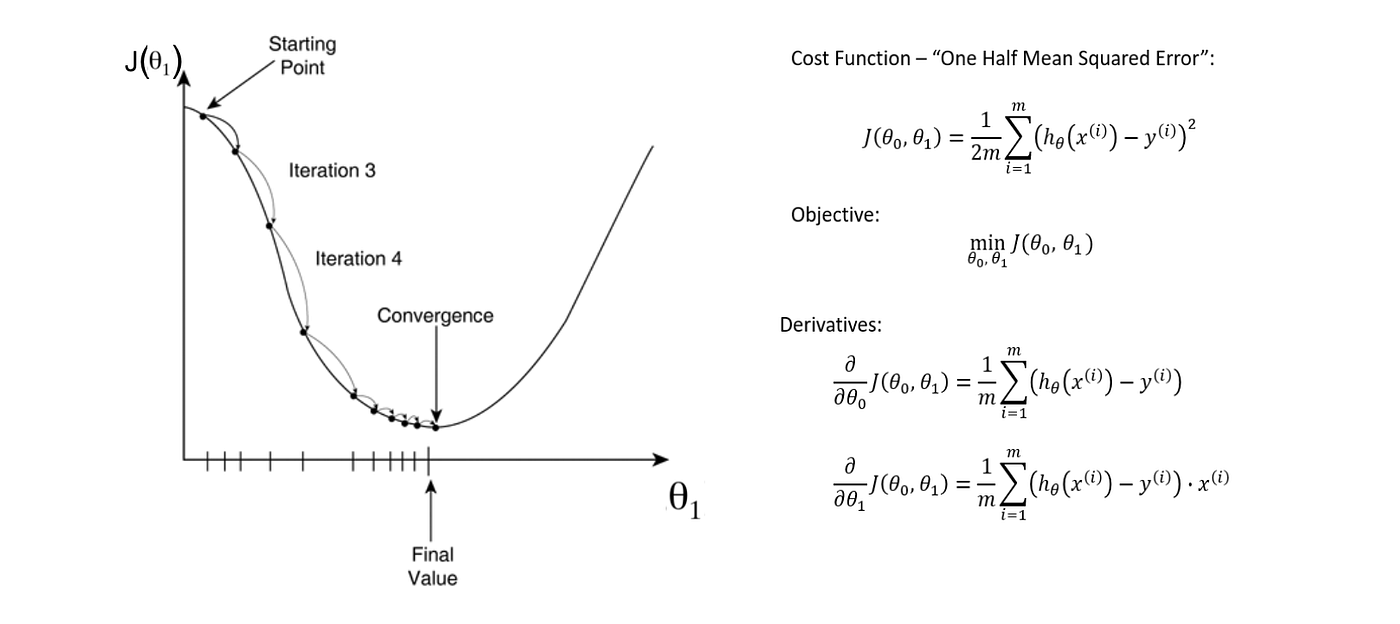θ1 gradually converges towards a minimum value.

The derivative of a function (in our case, J(θ)) on each parameter (in our case weight θ) tells us the sensitivity of the function with respect to that variable or how changing the variable impacts the function value. Gradient descent, thereforeenables the learning process to make corrective updates to the learned estimates that move the model toward an optimal combination of parameters (θ). The cost is calculated for a machine learning algorithm over the entire training dataset for each iteration of the gradient descent algorithm. In Gradient Descent, one iteration of the algorithm is called one batch, which denotes the total number of samples from a dataset that is used for calculating the gradient for each iteration.

## The step of the derivation

It would be better if you have some basic understanding of calculus because the technique of the partial derivative and the chain rule is being applied in this case.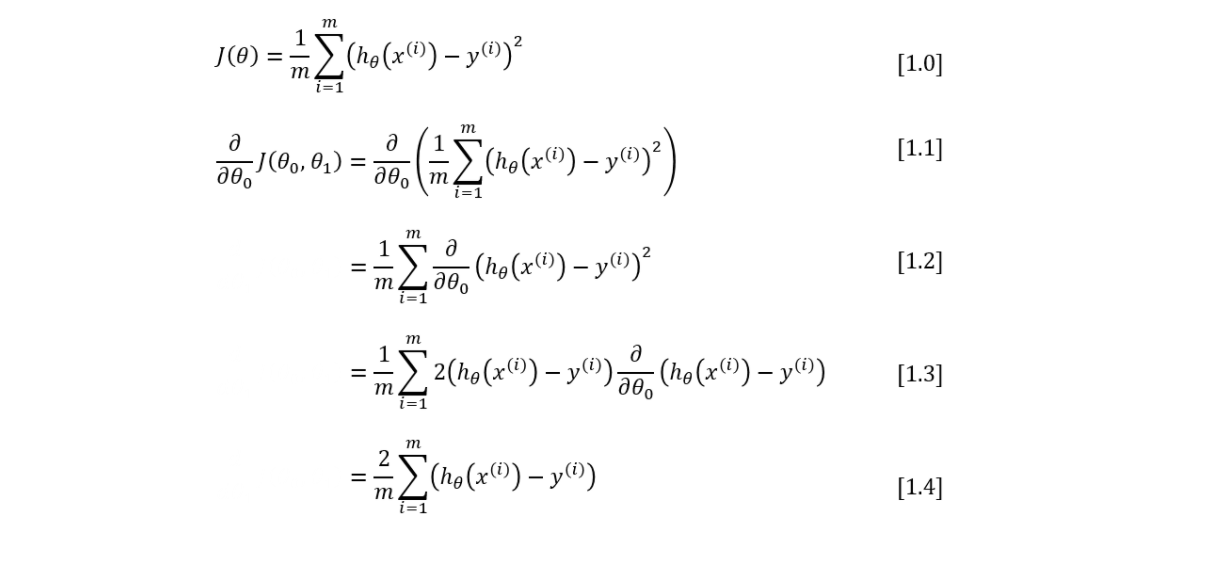To solve for the gradient, we iterate through our data points using our new weight ‘θ0 and bias ‘θ1’ values and compute the partial derivatives. This new gradient tells us the slope of our cost function at our current position (current parameter values) and the direction we should move to update our parameters. The size of our update is controlled by the learning rate.

## Learning rate (α)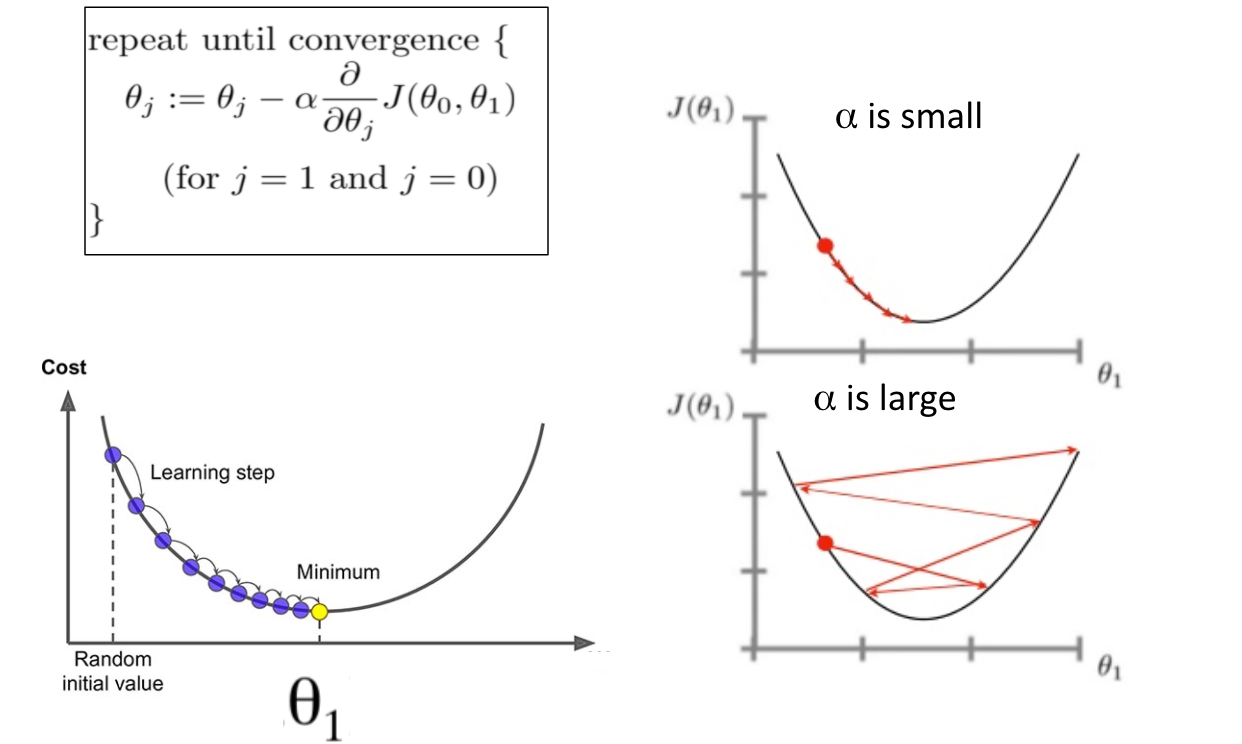Note that we used ‘:=’ to denote an assignment or an update.

The size of these steps is called the learning rate (α) that gives us some additional control over how large of steps we make. With a large learning rate, we can cover more ground each step, but we risk overshooting the lowest point since the slope of the hill is constantly changing. With a very low learning rate, we can confidently move in the direction of the negative gradient since we are recalculating it so frequently. A low learning rate is more precise, but calculating the gradient is time-consuming, so it will take us a very long time to get to the bottom. The most commonly used rates are: 0.001, 0.003, 0.01, 0.03, 0.1, 0.3.

Now let’s discuss the three variants of gradient descent algorithm. The main difference between them is the amount of data we use when computing the gradients for each learning step. The trade-off between them is the accuracy of the gradient versus the time complexity to perform each parameter’s update (learning step).

## Stochastic gradient descent (SGD)

However, there is a disadvantage of applying a typical Gradient Descent optimization technique in our dataset. It becomes computationally very expensive to perform because we have to use all of the one million samples for completing one iteration, and it has to be done for every iteration until the minimum point is reached. This problem can be solved by Stochastic Gradient Descent.

The word ‘stochastic’ means a system or a process that is linked with a random probability. Stochastic gradient descent uses this idea to speed up the process of performing gradient descent. Hence, unlike the typical Gradient Descent optimization, instead of using the whole data set for each iteration, we are able to use the cost gradient of only 1 example at each iteration (details are shown in the graph below). Even though using the whole dataset is really useful for getting to the minima in a less noisy or less random manner, the problem arises when our datasets get really large.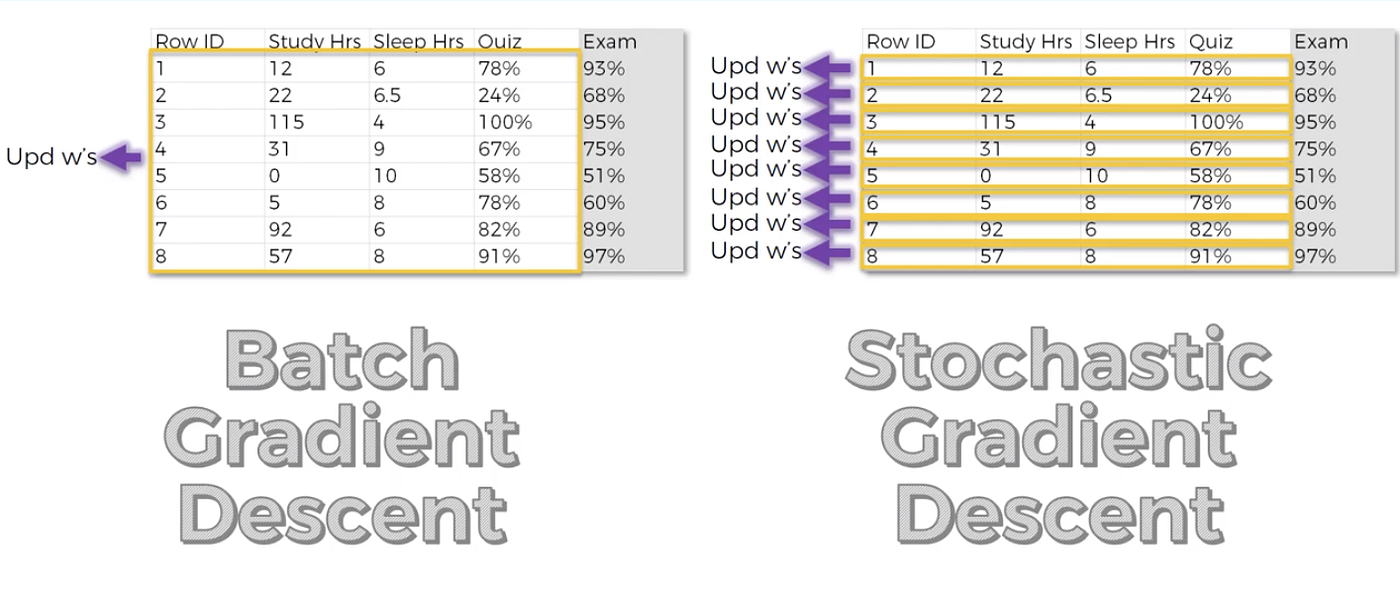The two main differences are that the stochastic gradient descent method helps us avoid the problem where we find those local extremities or local minimums rather than the overall global minimum.

As mentioned, the stochastic gradient descent method is doing one iteration or one row at a time, and therefore, the fluctuations are much higher than the batch gradient descent.

Three variants of gradient descent algorithm

• Batch gradient descent (BGD): calculate the error for each example in the training dataset, but only updates the model after all training examples have been evaluated.
• Stochastic gradient descent (SGD): calculate the error and updates the model for each examplein the training dataset.
• Mini-Batch gradient descent: split the training dataset into small batches that are used to calculate model error and updated model coefficients. (the most common implementation of gradient descent used in the field of deep learning)

Mini-Batch gradient descent can find a balance between the robustness of SGD and the efficiency of BGD.

# Summary

After reading this blog, you now should a better understanding of the 5 concepts of Gradient Descent and Cost Function:

• What is the Cost Function is and how to minimise it?
• How to calculate gradient descent?
• What is the learning rate?
• What is Batch Gradient Descent (BGD)?
• Why is Stochastic Gradient Descent (SGD) important in machine learning?

By subscribing you accept KDnuggets Privacy Policy

By subscribing you accept KDnuggets Privacy Policy

By subscribing you accept KDnuggets Privacy Policy